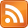# kaashif's blog

Programming, software freedom and Unix## What's a monoid?

### 2014-01-14

If you have spent time on programming or technology boards like /g/ or /r/programming, chances are you might have heard the word "monad" thrown around a lot. You may have even heard the oft misquoted phrase "A monad is a monoid in the class of endofunctors" intended to be a joke, or to scare programmers away from scary functional languages like Haskell. The truth is that monads aren't even slightly the same sort of thing in Haskell, but they do relate somewhat. Monoids are, in actual fact, very, very simple.

## Sets and operators

Let's take an example: suppose you have a set of things S and a single binary operator. This binary operator takes two things from your set and outputs a third thing, which is also in your set. There is no pair of operands for which the result is outside the set you started with - the set is closed. The set also contains an identity element - there exists an element which does not change other elements when combined with them, using your binary operator. The operator must also be associative, i.e. the order in which the operations are evaluated does not change the results. These laws are expressed more clearly as follows: $$S \circ S \to S$$ $$i \circ e = e \text{ for all } e \text{ in } S$$ $$a \circ (b \circ c) = (a \circ b) \circ c$$

We could make this an even more relatable example by using the set of real numbers and the addition operation, forming a monoid and satisfying the laws as follows: $$\mathbb{R} + \mathbb{R} \to \mathbb{R}$$ $$0 + e = e \text{ for all } e \text{ in } \mathbb{R}$$ $$a + (b + c) = (a + b) + c$$

This means that whenever you're adding real numbers (which we all do on a daily basis), you're utilising monoids. Notice that subtraction and division are not associative, so cannot form monoids. We aren't limited to sets of numbers either, you can form monoids from matrices, vectors and functions. Indeed, a set can contain any sort of structure, meaning monoids can be very general or very specific, depending on the sort of structures it contains. For example, defining a monoid of numbers and addition is useful in a small number of cases, while a monoid of functions and function composition is very useful in many cases. Monoids can be generalised, and the resulting generalisation will be very useful, generally.

## Generalising monoids

While it is all well and good having a single set and a single binary operator, which maps members of that one set to members of the same set, it would be useful to have a structure defined as an arbitrary number of sets and an arbitrary number of functions. We could define such a structure as consisting of the following:

• A set of sets
• A set of functions mapping sets to other sets

In some cases, rather than having sets of sets within our structure, we may want to use magmas or semigroups, or something unrelated to groups entirely. For this reason, it is better to say that our new structure contains a set of objects, and morphisms between these objects. "Object" is a term which refers to any algebraic structure, and "morphism" refers to any possible mapping between these objects. So now, our structure consists of:

• A set of objects
• A set of morphisms mapping objects to other objects

This structure is not all that different to a monoid, and it becomes very obvious if we say a monoid merely consists of:

• A singleton, containing a single set (e.g. all real numbers, all functions)
• A single morphism (e.g. addition, function composition)

Since there is only one object, the morphism can only map the object to itself, thus is always an endomorphism. We can also think about the binary operation (e.g. addition) as a set of unary operations (e.g, add 1, add 2, add 3), and see the monoid as a set S, and a set of unary functions equal in order to S. We get the same result whether we think about a single endomorphism or multiple, so it doesn't really matter.

What we are slowly working towards, as our definitions get progressively more general, is a category. Categories are incredibly useful and can become so general that they are used to formalise all of mathematics. They actually have uses too, such as considering the objects as representing types in a programming language, and the morphisms as representing functions.